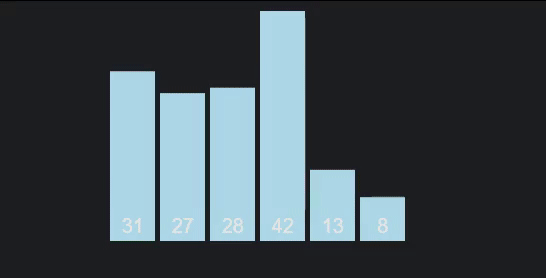# Understanding Quick Sort via JavaScript

Published on February 14, 2020By Joshua Hall
Developer and author at DigitalOcean.While we believe that this content benefits our community, we have not yet thoroughly reviewed it. If you have any suggestions for improvements, please let us know by clicking the “report an issue“ button at the bottom of the tutorial.

One problem of working with merge sorts is that they need to create and store so many arrays in memory with mostly the redundant data. If we’re limited on memory, we can resort to a quick sort to run it “in place”, meaning the changes and results all happen directly with what’s being sorted, thus saving on memory.

## Prerequisites

We’re going to be going over the standard recursive version, although you can do this iteratively, so understanding how recursion works will be helpful, which you can brush up on here.

## Concept

Quick sort is definitely one of the less intuitive algorithms, so here’s a very simple overview.

We select a number, called our `pivot`, which we’ll compare every number to when we loop through our items. The goal is to reorganize the array so it is partitioned into two halves, with everything in each either being less than or greater than our pivot. When the pivot is in it’s final position we’ll move on to doing the same thing with a new pivot, with every pivot being cemented in place until every item has been a pivot at least once.Graphic/Animation thanks to VisuAlgo.net

Like merge sort, the complexity on average is O(nlogn), so it’s up to you which you prefer.

## Practice Data

As always, here’s an unsorted array of 50 random numbers to play with. I also recommend looking into JavaScript’s performance api to compare it to other algorithms and with different data.

``````const unsortedArr = [31, 27, 28, 42, 13, 8, 11, 30, 17, 41, 15, 43, 1, 36, 9, 16, 20, 35, 48, 37, 7, 26, 34, 21, 22, 6, 29, 32, 49, 10, 12, 19, 24, 38, 5, 14, 44, 40, 3, 50, 46, 25, 18, 33, 47, 4, 45, 39, 23, 2];
``````

## Pivot

Firstly, we’ll need our pivot utility function. There’s 4 main parts to this, our swapper, the loop, the pivot itself, and our pointer.

Our pointer is just a placeholder for our pivot. Every time our loop progresses, each item is compared to the pivot and if it is smaller it’s swapped with our pointer. Every time we do this is to ensure that the pointer is ahead of everything smaller than the pivot and before everything that’s larger. When everything is complete, and our array is partitioned, then we can assign the pivot to the pointer’s index as its final position.

Our swap works by just reassigning the indexes of each item with each other’s index, nothing too crazy.

``````const pivot = (arr, start = 0, end = arr.length + 1) => {
const swap = (list, a, b) => [list[a], list[b]] = [list[b], list[a]];

let pivot = arr[start],
pointer = start;

for (let i = start; i < arr.length; i++) {
if (arr[i] < pivot  ) {
pointer++;
swap(arr, pointer, i);
}
};
swap(arr, start, pointer);

return pointer;
}
``````

## Quick Sort

Implementation is simultaneously pretty simple and a bit confusing, as recursion tends to be. We’re going to use our `pivot` function to get the first sorted item from our array. With that, we’ll recursively run our `quickSort` to do the same process on the first half of our partitioned array, which will repeat until there’s nothing left to sort, then do the same for the other half. When both are done our fully sorted array can be returned.

``````const quickSort = (arr, start = 0, end = arr.length) => {
let pivotIndex = pivot(arr, start, end);

if (start >= end) return arr;
quickSort(arr, start, pivotIndex);
quickSort(arr, pivotIndex + 1, end);

return arr;
};

console.log(quickSort(unsortedArr));
``````

## Conclusion

Quick sort was definitely one of the most difficult sorting algorithm to wrap my head around. Between having 4 changing parts and 2 recursion stacks, this is definitely something you probably shouldn’t expect to remember perfectly and may want to bookmark for later reference.Developer and author at DigitalOcean.

#### Still looking for an answer?

You can type !ref in this text area to quickly search our full set of tutorials, documentation & marketplace offerings and insert the link!

A little fix for the quicksort function:

``````const quickSort = (arr, start = 0, end = arr.length) => {

if (start >= end) return arr;//conditional statement comes before we get the new pointer position to avoid getting undefined.

let pivotIndex = pivot(arr, start, end);

quickSort(arr, start, pivotIndex);
quickSort(arr, pivotIndex + 1, end);

return arr;
};

console.log(quickSort(unsortedArr));
``````

The above solution is not working with [10, 7, 8, 9, 1, 5] when we take pivot as last element

Hey Josh, could you explain the stopping condition in the recursion?

``````if (start >= end) return arr;
``````

that would help a lot. thanks

Looks like the `pivot` function doesn’t actually use the `end` variable that’s past in. As a result, if the `start` is always near or at the beginning of the list this method will traverse the entire list each time causing a hit to this algorithms’ performance.

Minor updates to the code provided works as expected within the bounds passed to the pivot function:

``````const pivot = (arr, start, end) => {
const swap = (list, a, b) => [list[a], list[b]] = [list[b], list[a]];

let pivot = arr[start],
pointer = start;

for (let i = start; i < end; i++) {
if (arr[i] < pivot) {
pointer++;
swap(arr, pointer, i);
}
};
swap(arr, start, pointer);

return pointer;
}

const quickSort = (arr) => {
quickSortHelper(arr, 0, arr.length)

return arr;
};

function quickSortHelper(arr, start, end) {
let pivotIndex = pivot(arr, start, end);

if (start >= end) return arr;
quickSortHelper(arr, start, pivotIndex);
quickSortHelper(arr, pivotIndex + 1, end - 1);
}
``````